# Division Printable primary worksheets

All of our division resources come complete with answer sheets to save time for busy teachers and parents.Input your child’s scores and keep track of your child's progress.

Filters

### Balancing equations - Multiplication and division

Students are asked to work out the sums by finding the value of each letter.

Age: 10 - 11
1
2
3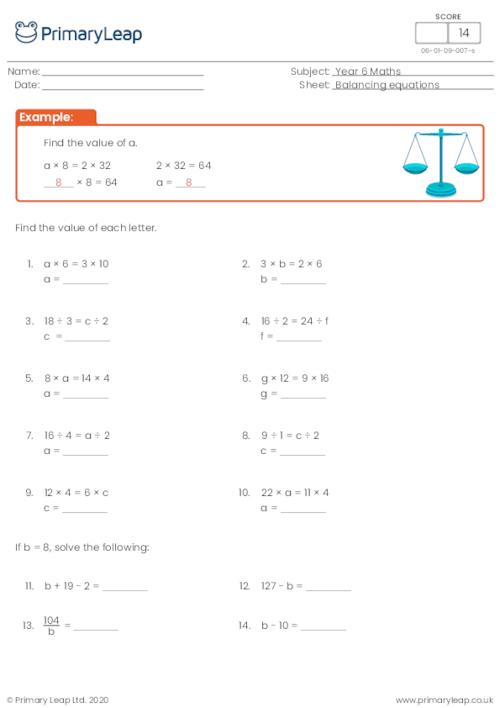### Balancing Equations - Multiplication and division

Maths: Students are asked to work out the sums. This resource will help with multiplication and division.

Age: 9 - 10
1
2
3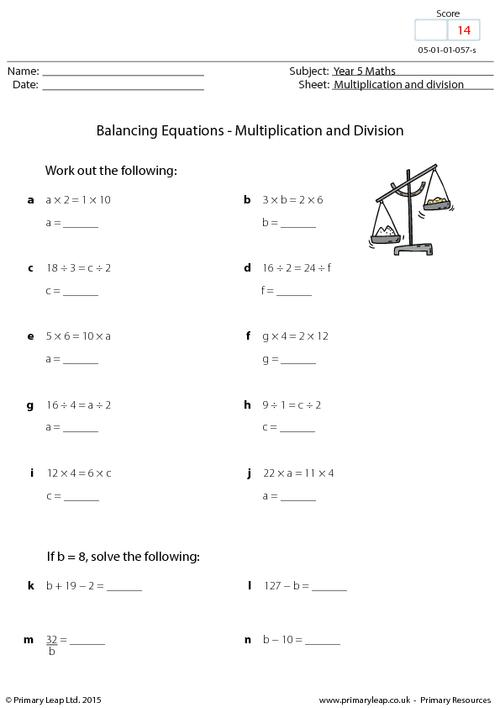### Solving the Equation - Multiplication and division

Maths: Students are asked to work out the value of each letter to complete the sum. There are 14 sums to work out.

Age: 8 - 9
1
2
3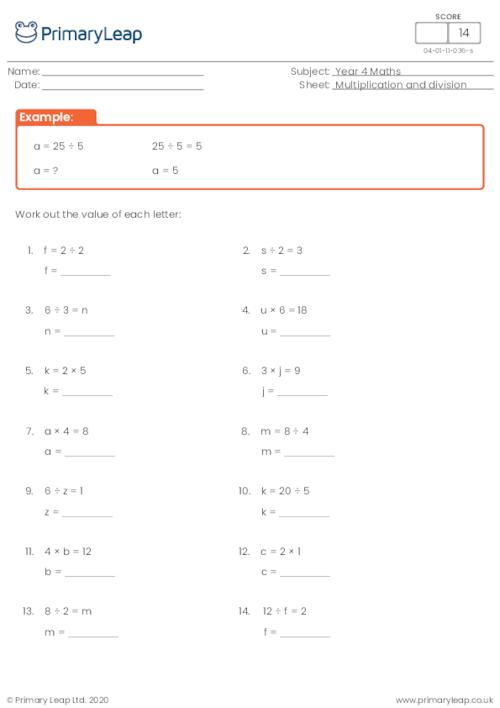### Division sentence by 5

A KS2 maths resource on division sentences.

Age: 7 - 8
1
2
3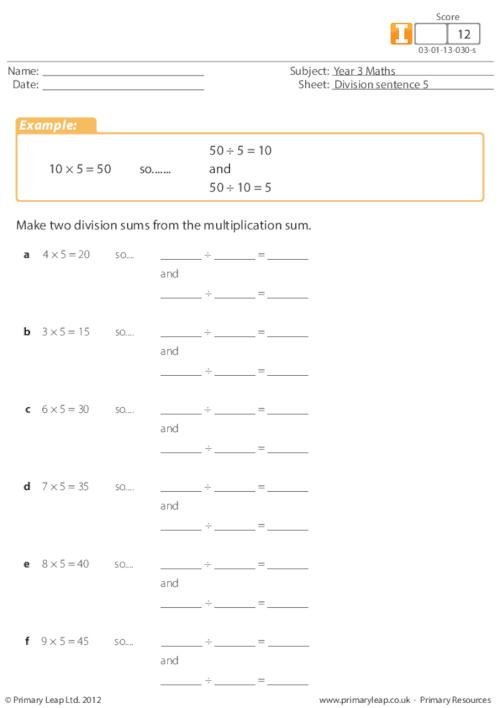### Division sentence by 3

A division sentence maths resource for KS2 pupils.

Age: 7 - 8
1
2
3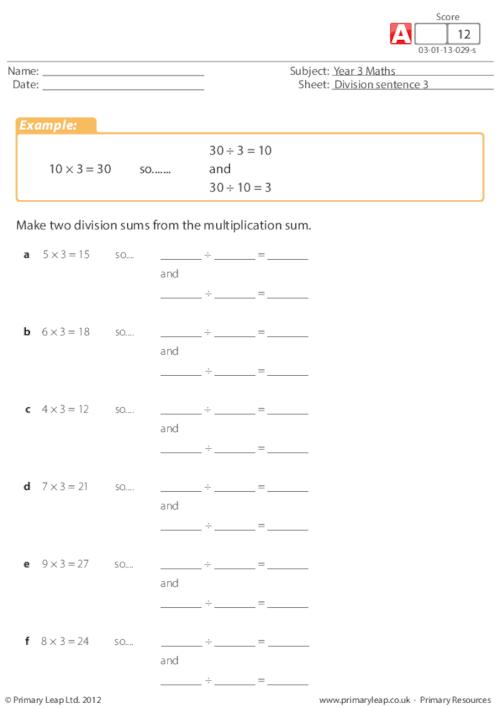### Division sentence by 2

A year 3 primary resource on division sentences.

Age: 7 - 8
1
2
3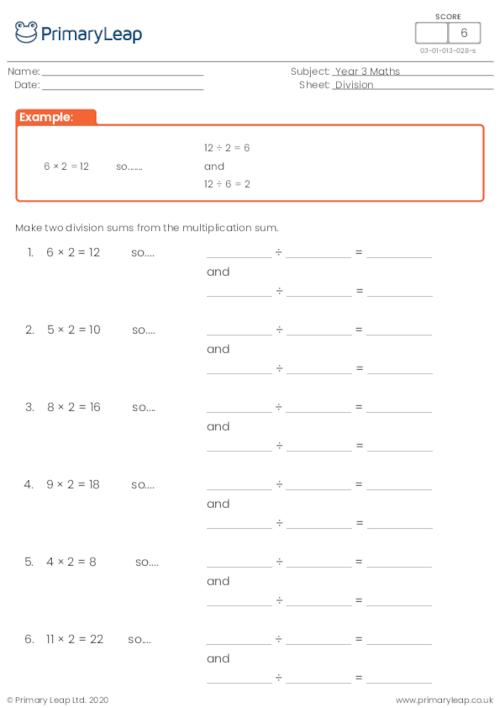### Division

Math: Division - Answer the two sets of division questions.

Age: 8 - 9
1
2
3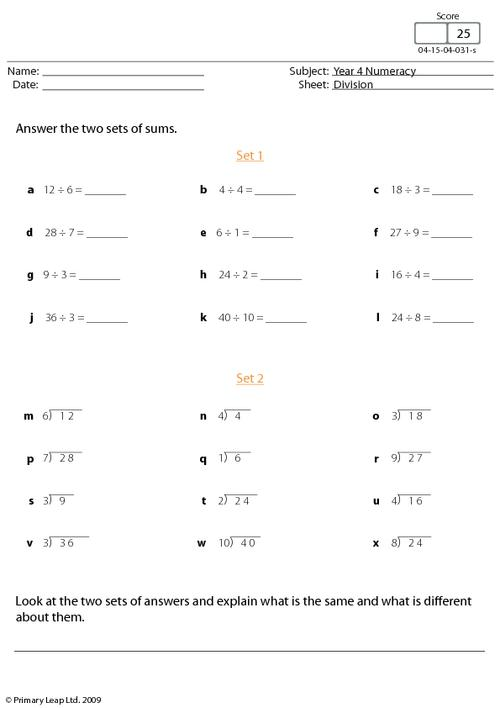### Division: 6 digits by 2

Math: Division - Dividing 6 digits by 2.

Age: 10 - 11
1
2
3### Division: 5 digits by 1 - set 5

Math: Division - 21 division questions dividing 5 digits by 1.

Age: 9 - 10
1
2
3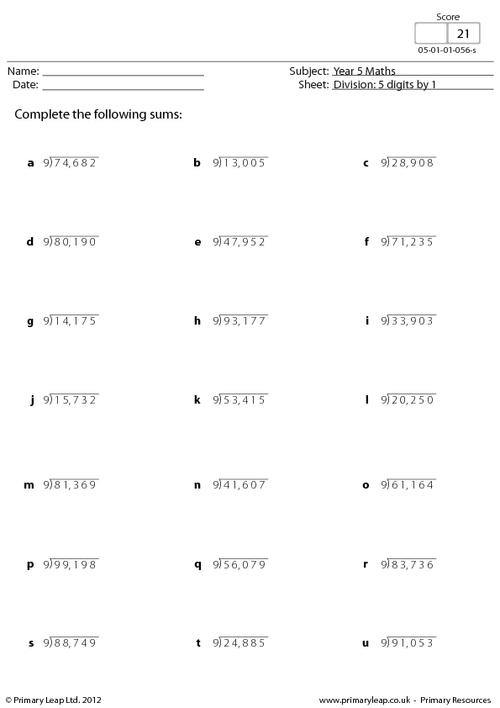### Division: 5 digits by 1 - set 4

Math: Division - 21 division questions dividing 5 digits by 1.

Age: 9 - 10
1
2
3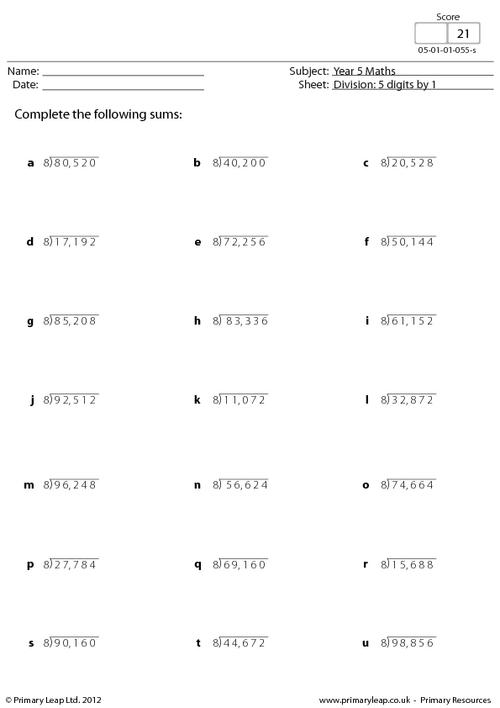### Division: 5 digits by 1 - set 3

Math: Division - 21 division questions dividing 5 digits by 1.

Age: 9 - 10
1
2
3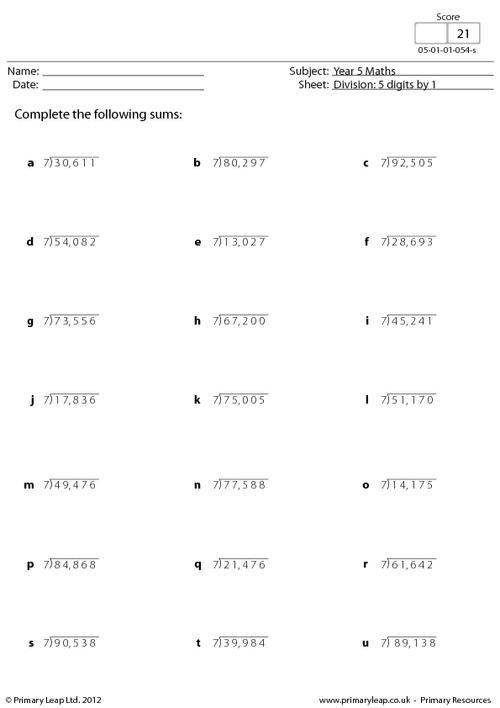### Division: 5 digits by 1 - set 2

Math: Division - 21 division questions dividing 5 digits by 1.

Age: 9 - 10
1
2
3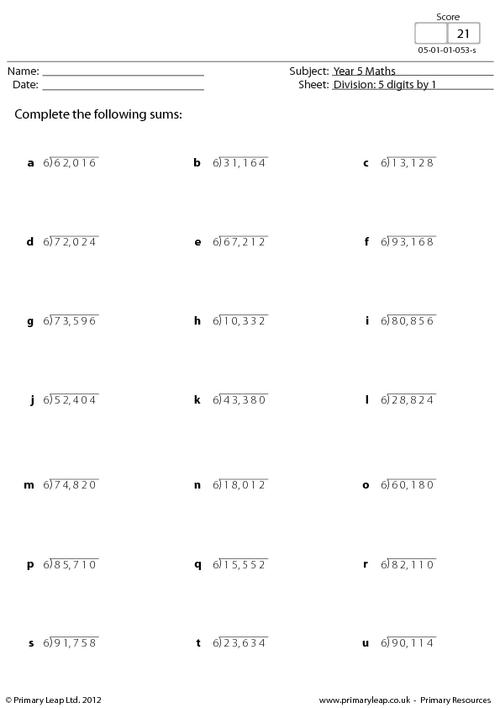### Division: 5 digits by 1 - set 1

Math: Division - 21 division questions dividing 5 digits by 1.

Age: 9 - 10
1
2
3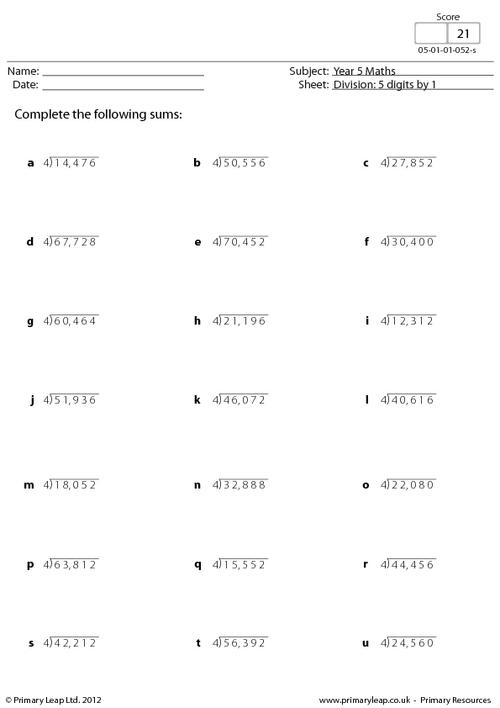### Division: 4 digits by 1 - set 7

Year 4 Maths: Division - This worksheet asks children to divide four-digit numbers by 9. (21 questions)

Age: 8 - 9
1
2
3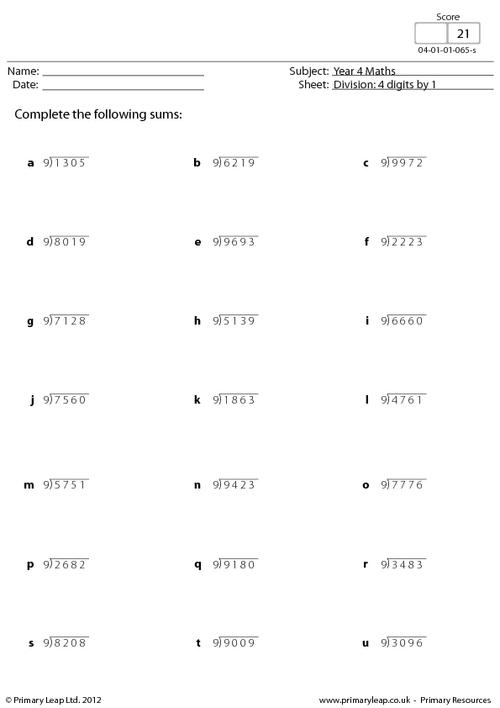### Division: 4 digits by 1 - set 6

Year 4 Maths: Division - This worksheet asks children to divide four-digit numbers by 8. (21 questions)

Age: 8 - 9
1
2
3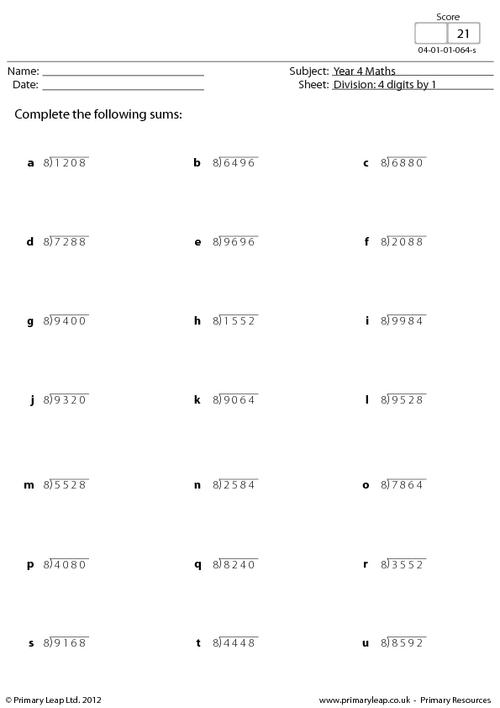### Division: 4 digits by 1 - set 5

Year 4 Maths: Division - This worksheet asks children to divide four-digit numbers by 7. (21 questions)

Age: 8 - 9
1
2
3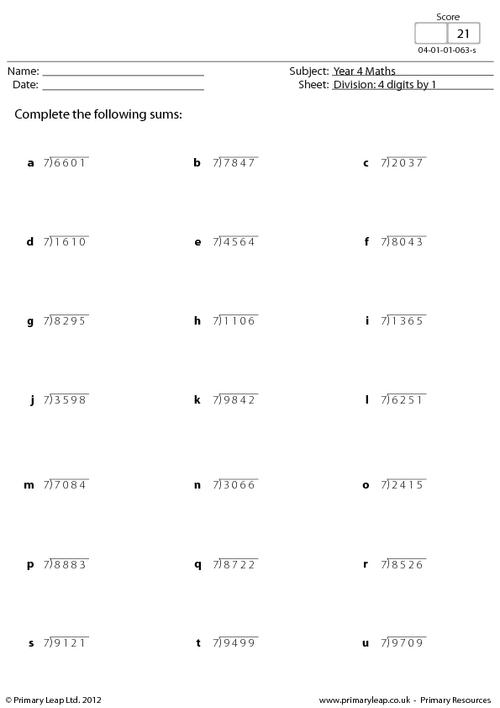### Division: 4 digits by 1 - set 4

Year 4 Maths: Division - This worksheet asks children to divide four-digit numbers by 6. (21 questions)

Age: 8 - 9
1
2
3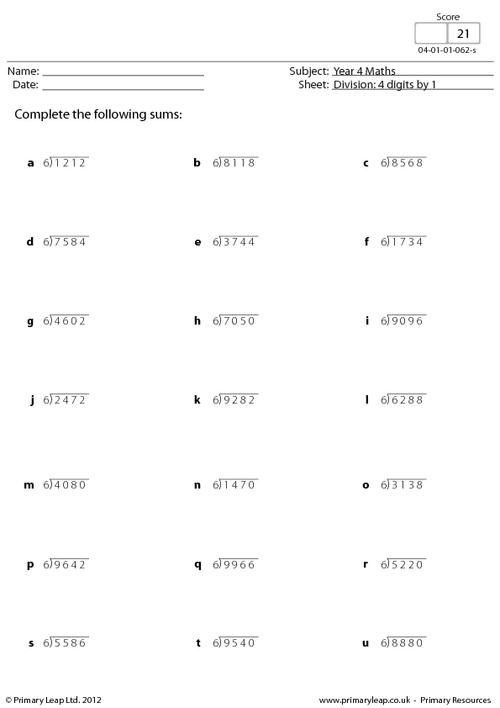### Division: 4 digits by 1 - set 3

Year 4 Maths: Division - This worksheet asks children to divide four-digit numbers by 5. (21 questions)

Age: 8 - 9
1
2
3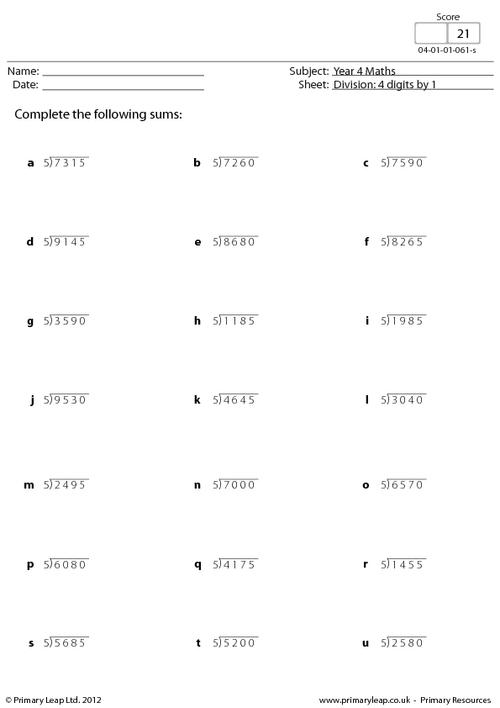### Division: 4 digits by 1 - set 2

Year 4 Maths: Division - This worksheet asks children to divide four-digit numbers by 4. (21 questions)

Age: 8 - 9
1
2
3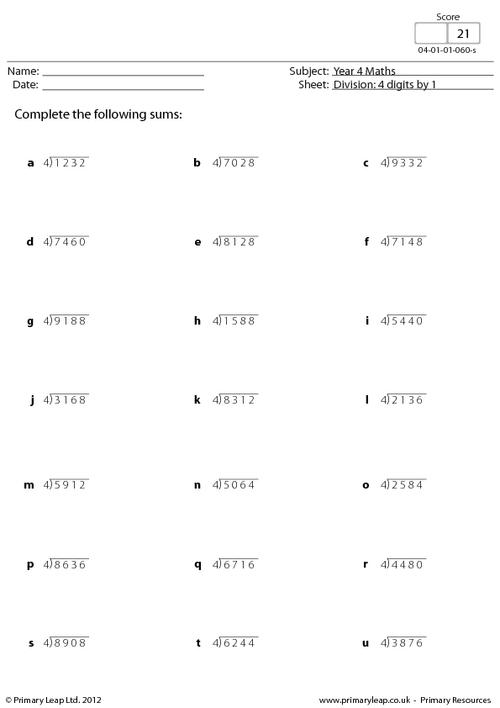### Division: 4 digits by 1 - set 1

Year 4 Maths: Division - This worksheet asks children to divide four-digit numbers by 3. (21 questions)

Age: 8 - 9
1
2
3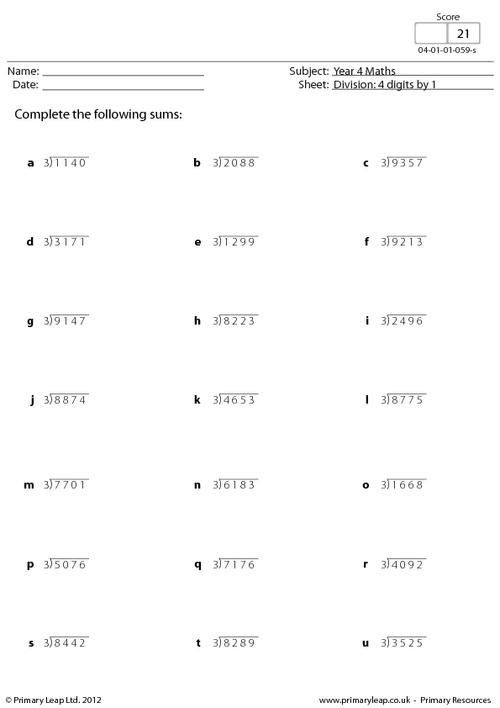### Related facts - Division by 12

Math: Division - These 8 division questions show how the same sum can be written in different ways/divide by 12.

Age: 9 - 10
1
2
3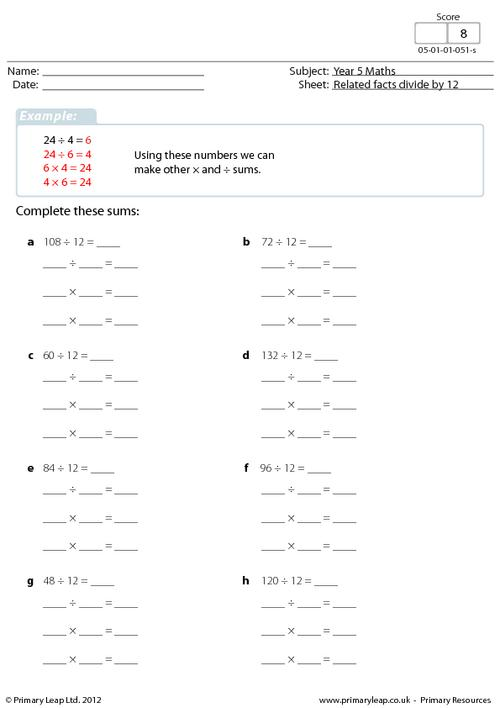### Related facts - Division by 11

Math: Division - These 8 division questions show how the same sum can be written in different ways/divide by 11.

Age: 9 - 10
1
2
3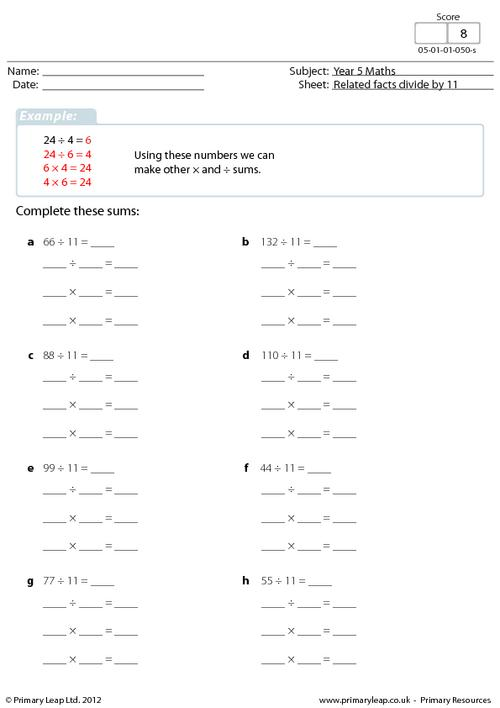### Related facts - Division by 10

Math: Division - These 8 division questions show how the same sum can be written in different ways/divide by 10.

Age: 9 - 10
1
2
3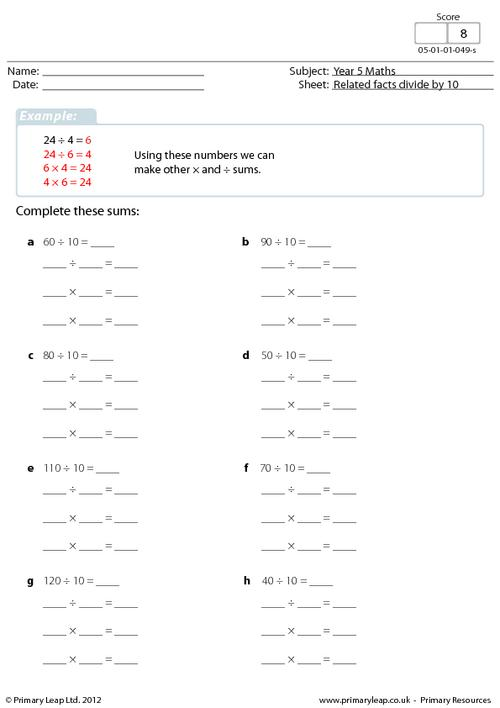### Related facts - Division by 9

Math: Division - These 8 division questions show how the same sum can be written in different ways/divide by 9.

Age: 9 - 10
1
2
3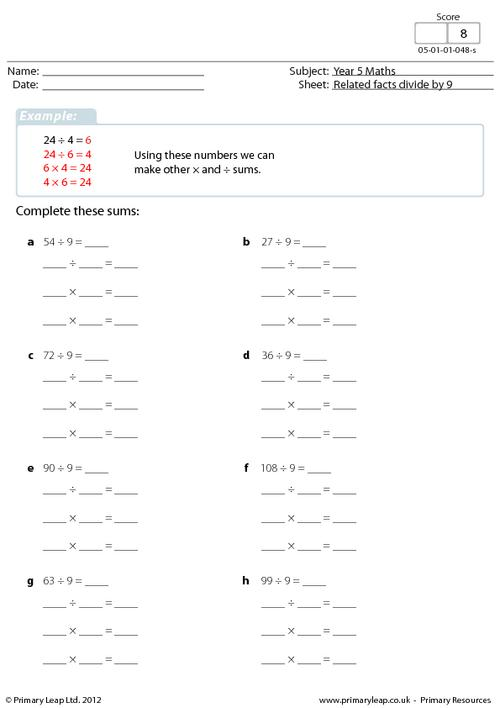### Related facts - Division by 8

Math: Division - These 8 division questions show how the same sum can be written in different ways/divide by 8.

Age: 9 - 10
1
2
3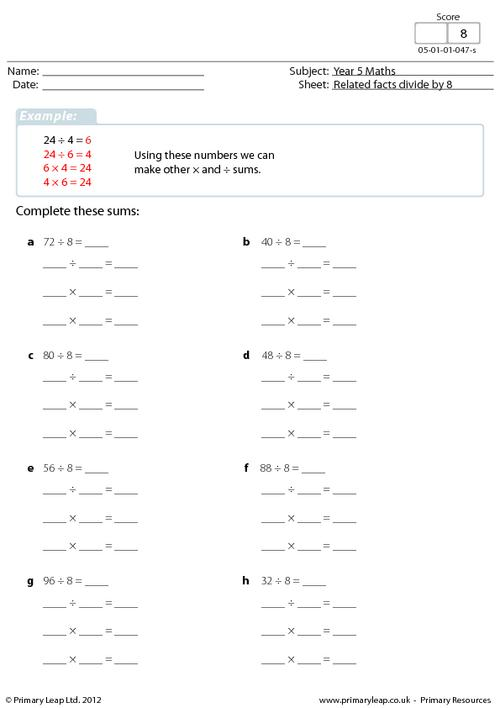### Related facts - Division by 7

Math: Division - These 8 division questions show how the same sum can be written in different ways/divide by 7.

Age: 9 - 10
1
2
3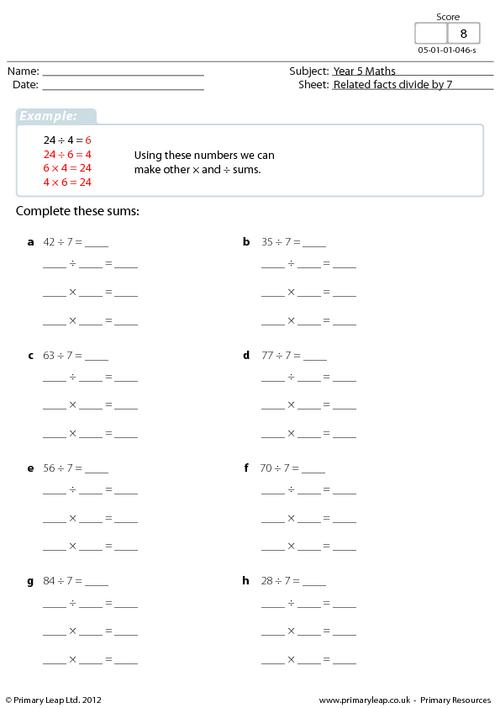### Related facts - Division by 6

Math: Division - These 8 division questions show how the same sum can be written in different ways/divide by 6.

Age: 9 - 10
1
2
3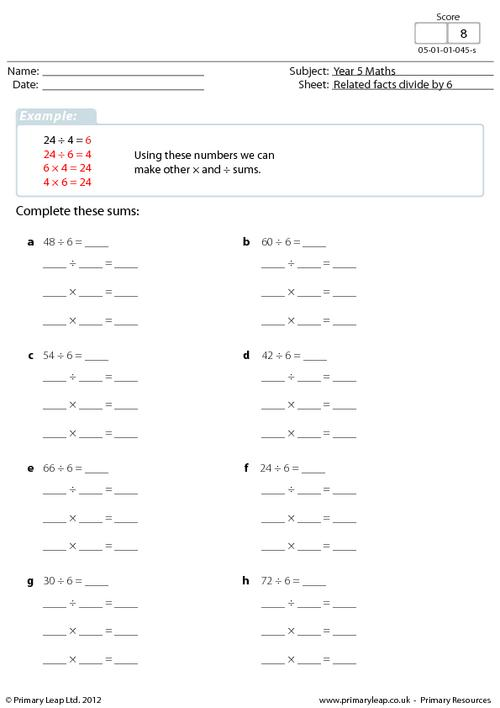### Related facts - Division by 5

Math: Division - These 8 division questions show how the same sum can be written in different ways/divide by 5.

Age: 9 - 10
1
2
3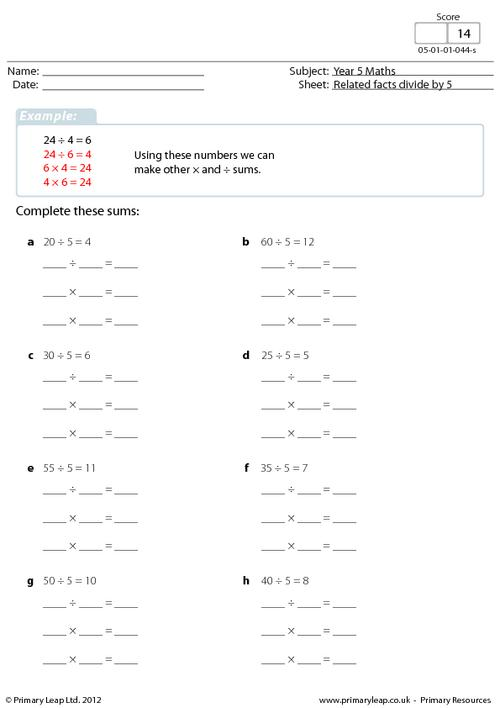### Short division with remainders 1

Math: Division - Solve the division sums with 2's and 5's/with remainders.

Age: 9 - 10
1
2
3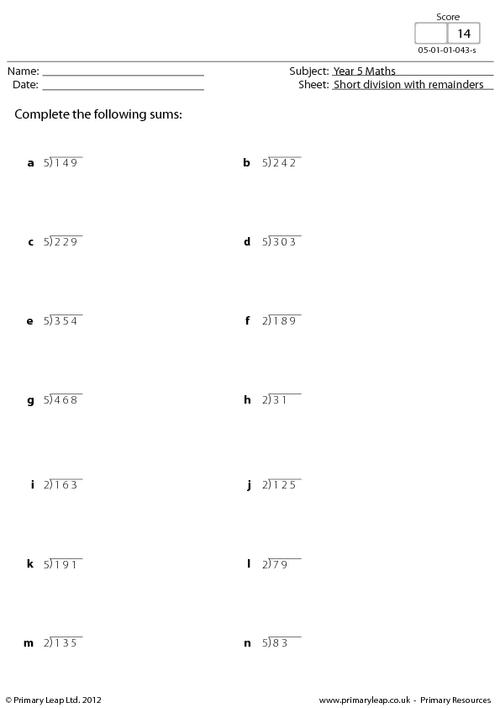### Short division 2

Math: Division - Solve the division facts with 2's and 5's/with remainders.

Age: 9 - 10
1
2
3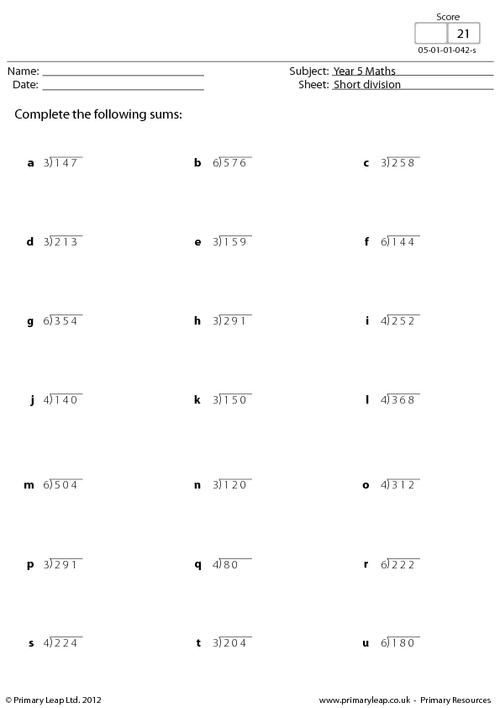### Short division

Math: Division - Solve the division facts with 7's, 8's and 9's/no remainders.

Age: 9 - 10
1
2
3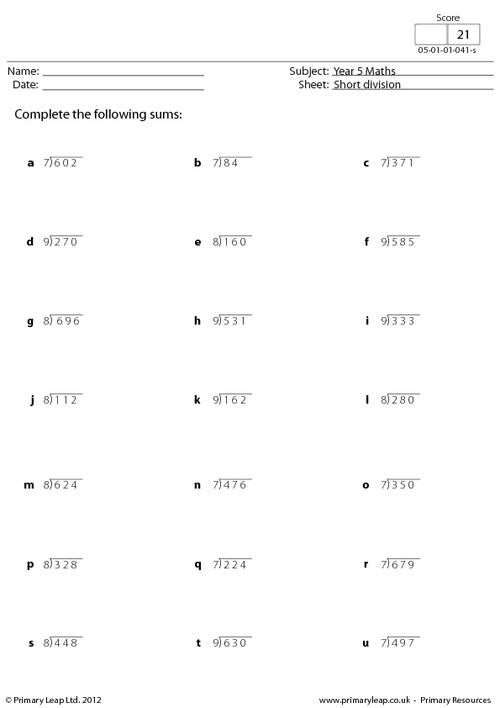### Division by 10

An advanced key stage one worksheet on dividing by ten. After completing this worksheet the child should have a better understanding of dividing by ten.

Age: 5 - 6
1
2
3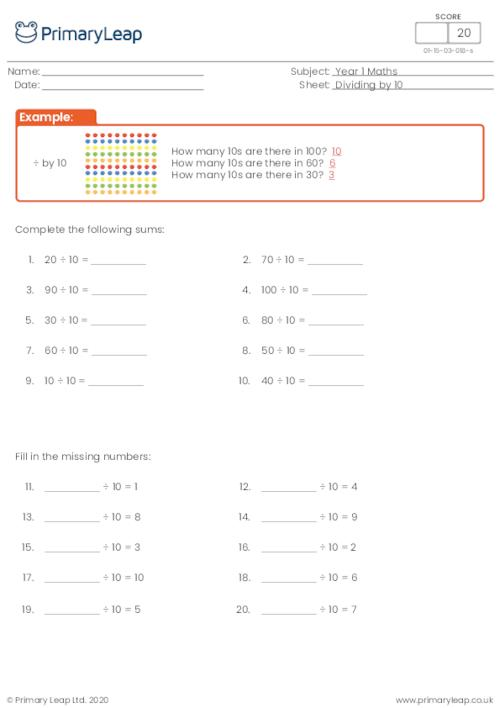### Division by 10

A year one worksheet introducing dividing by ten and shows two ways we can word division.

Age: 5 - 6
1
2
3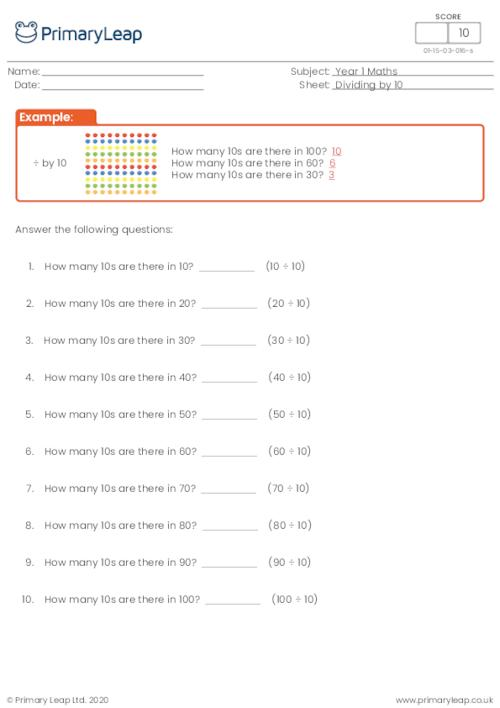### Division by 10

A year one worksheet on multiplying and dividing by ten. This primary resource will help children understand the relationship between dividing and multiplying.

Age: 5 - 6
1
2
3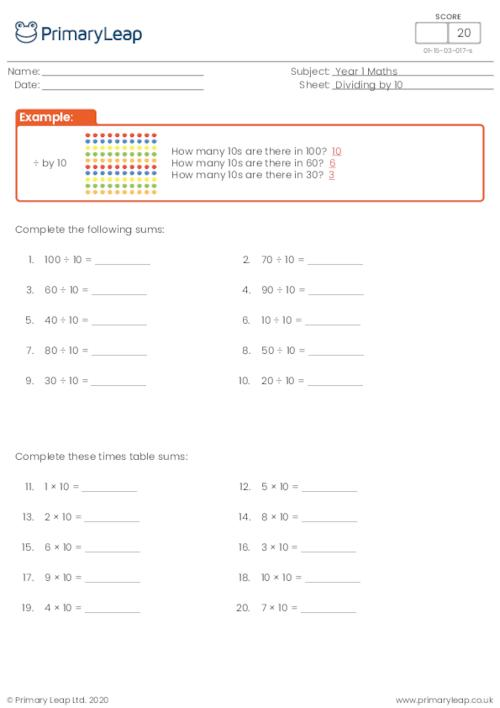### Division by 5

An advanced key stage one worksheet on dividing by five. After completing this worksheet the child should fully understand this concept.

Age: 5 - 6
1
2
3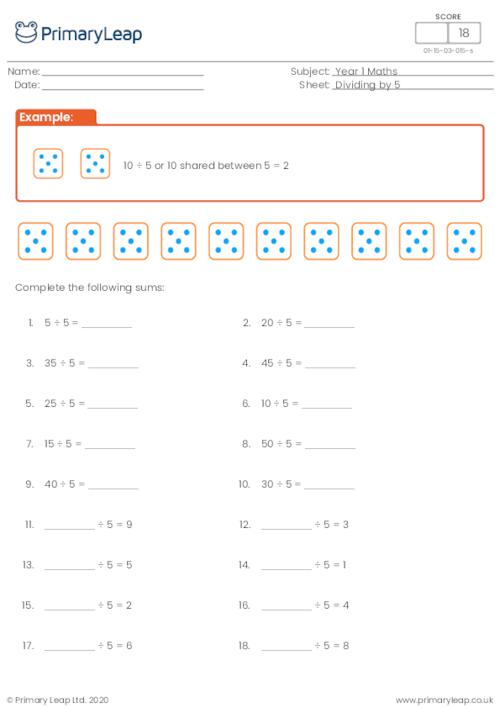### Division by 5

A year one primary resource on dividing by five. Division and multiplication are both important numeracy skills.

Age: 5 - 6
1
2
3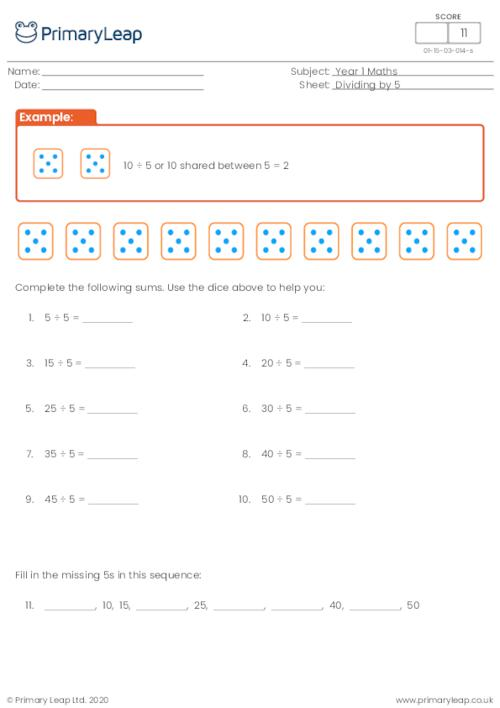### Division by 5

An introduction to dividing by five. This year one worksheet uses dice the help the pupil complete the worksheet.

Age: 5 - 6
1
2
3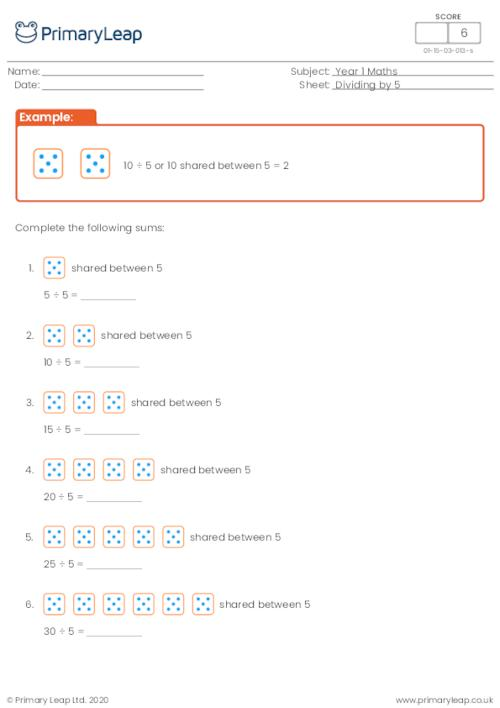### Division by 2

A year one primary resource on dividing by two. This worksheet has sweets to help the pupil understand the concepts of division.

Age: 5 - 6
1
2
3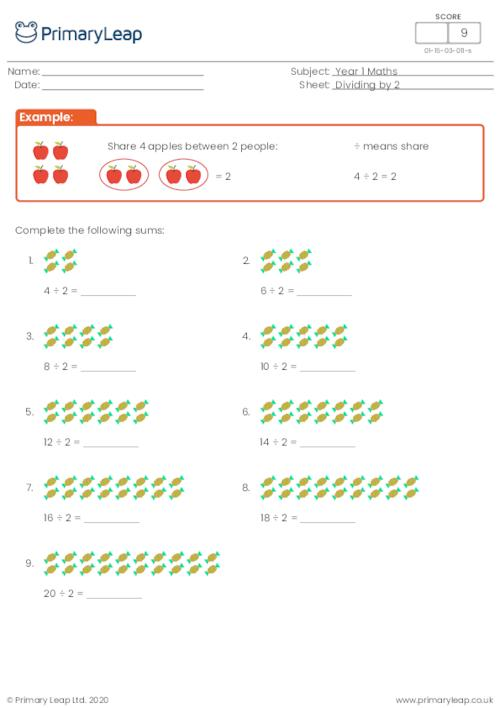### Division by 2

An advanced key stage one worksheet on dividing by two. Division is an important part of primary level numeracy.

Age: 5 - 6
1
2
3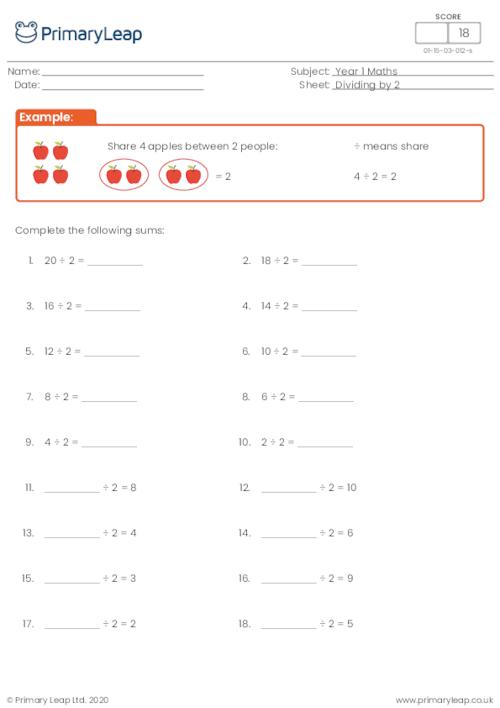### Division by 2

A year one worksheet on division by two. This worksheet has sweets to help the pupil share or divide by two.

Age: 5 - 6
1
2
3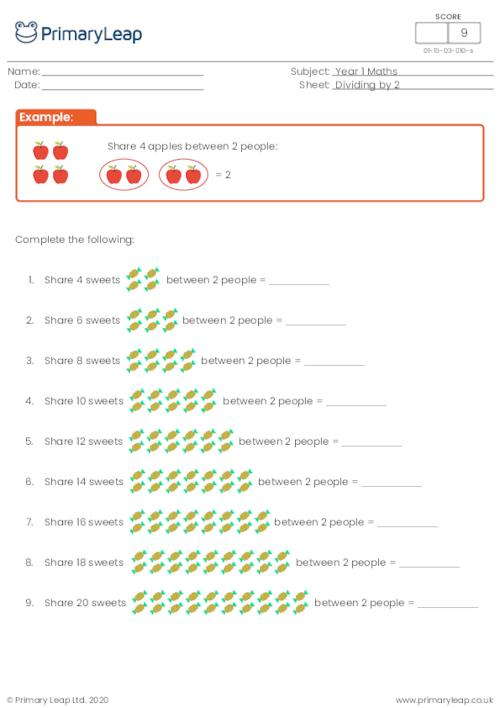### Division

Age: 8 - 9
1
2
3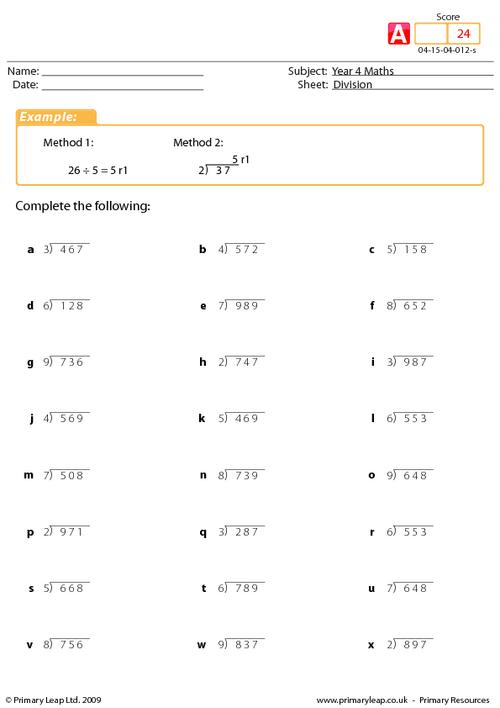### Division

Age: 8 - 9
1
2
3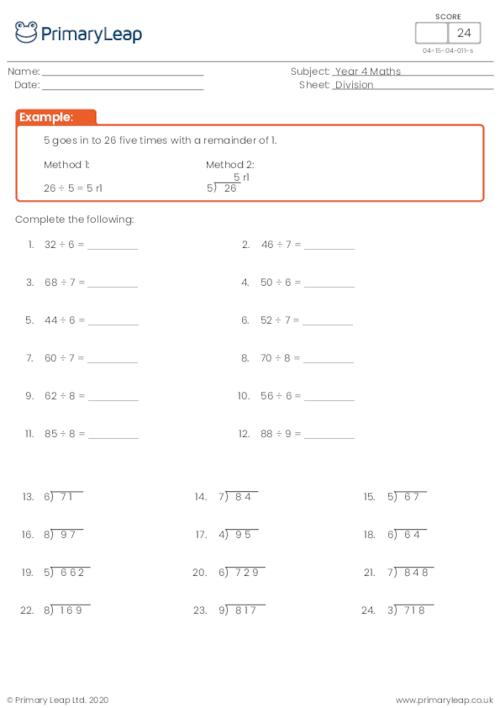### Division

Age: 8 - 9
1
2
3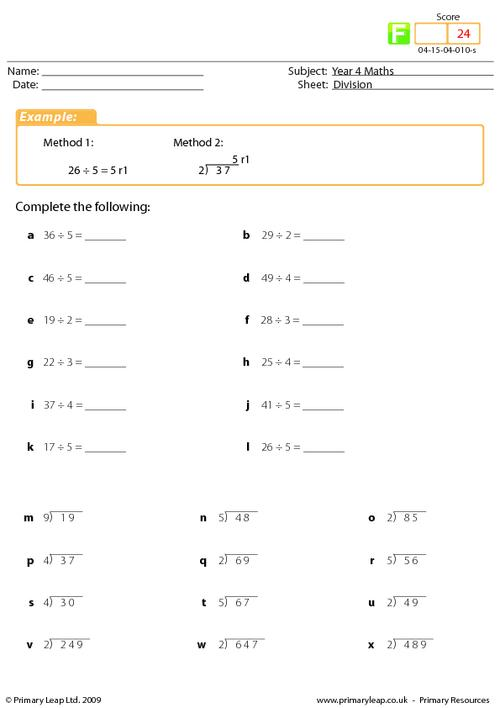### Simple division

A numeracy worksheet for key stage 2 on simple division. All the sums on this worksheet divide exactly and no remainders.

Age: 7 - 8
1
2
3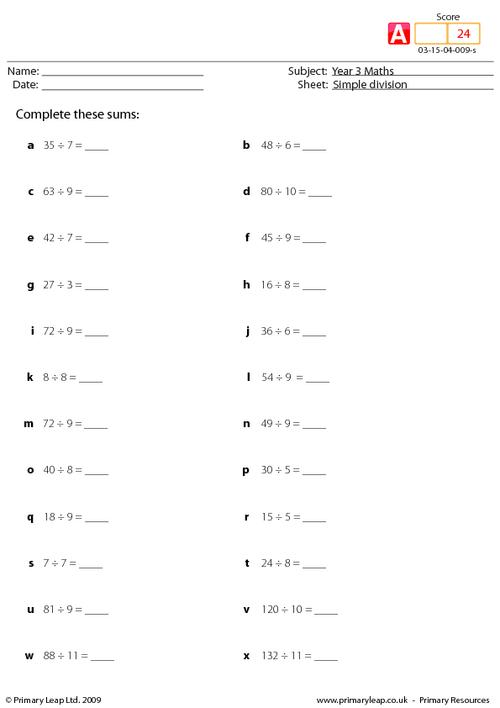### Simple division

Age: 7 - 8
1
2
3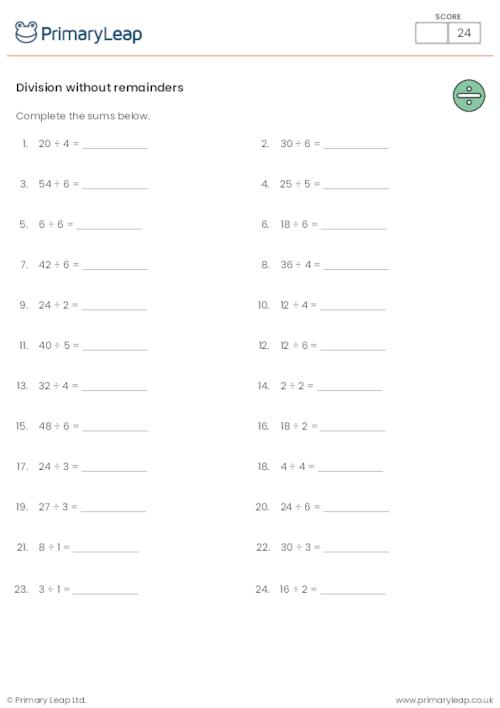### Simple division

Age: 7 - 8
1
2
3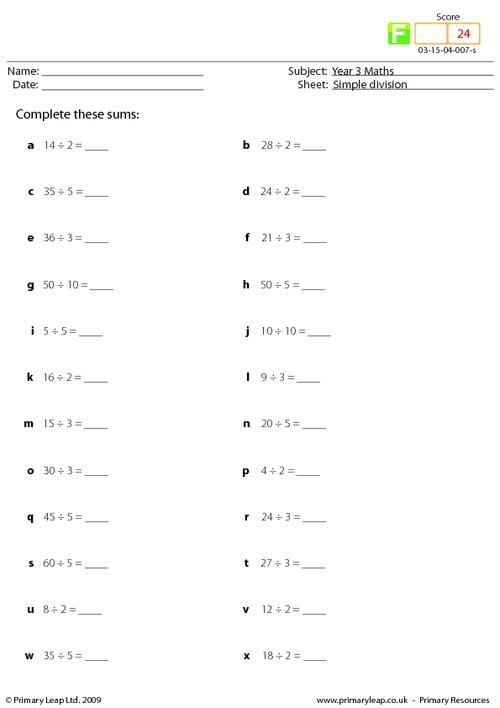### Division review

Year 2 maths - Revision worksheet including mixed questions on division.

Age: 6 - 7
1
2
3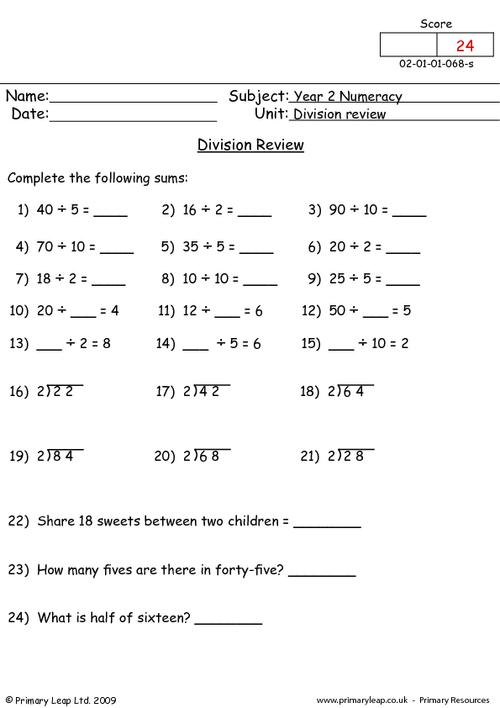### Division review

This primary resource for year one is a test of the pupils knowledge and understanding of dividing by two and ten. This is an excellent test of your child´s knowledge in this mathematical concept.

Age: 5 - 6
1
2
3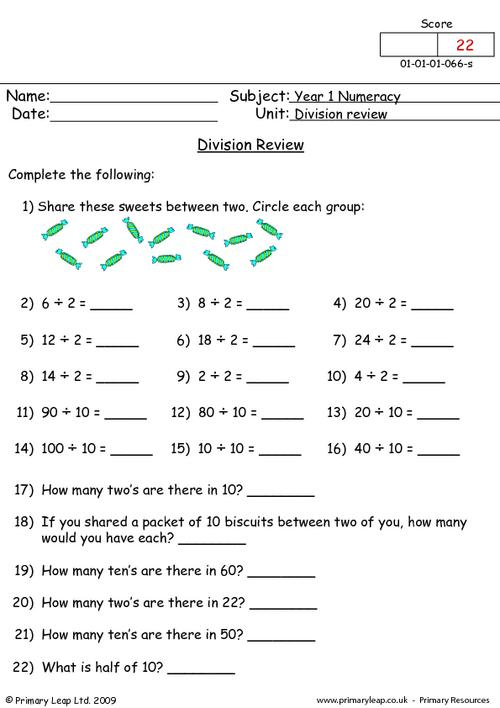of 2## division Worksheets - Created by teachers and loved by kids

Check out our ready-to-use division worksheets that can be used in the classroom or at home. Simply download or print and watch your child’s confidence grow with our easy to follow division printables.Learn

One of the best things about printable worksheets is that they make learning fun and easy. Explore our educational resources based on division and keep your children learning through these fun and engaging exercises.Progress

Our division printable worksheets are designed for progressive learning. Our engaging content enables a child to learn at their own speed and gain confidence quickly. When we help a child believe in themselves, we empower them for life.Improve